## Colored Tetriamond Parallelograms

Among the one-sided tetriamonds there are two parallelograms. To distinguish the parallelograms I rotate them so that two sides are horizontal and the other ones have a positive slope. If a is the length of the horizontal sides and b the length of the other ones the parallelogram gets the label a x b.This way the above pieces are 2x1-tetriamonds and 1x2-tetriamonds. Now we can colors the the six edge units and explore different sets:
• Sets of one-sided 2x1-parallelograms
• Sets of two-sided 2x1-parallelograms
• United sets of one-sided 2x1 and 1x2 parallelograms
For constructions with one-sided 1x2-tetriamonds you must only mirror the constructions with one-sided 2x1-tetriamonds. The set of two-sided pieces has the same number of elements as the set of one-sided pieces, but there are some constructions, which are only possible using the two-sided pieces. An example is a hexagonal ring of size 5 with a hexagonal hole of size 1.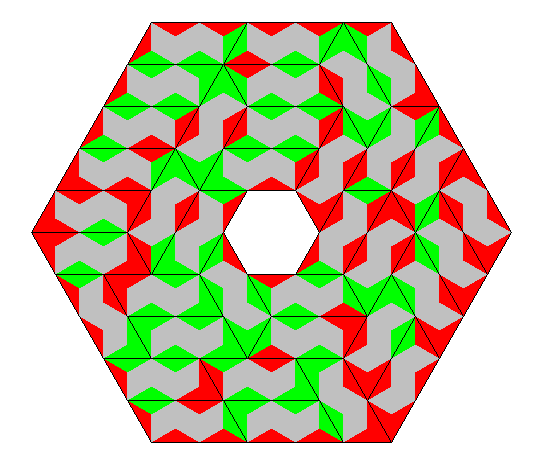Click the numbers of pieces to see some constructions.

Colors Parallelogram Type Number of Pieces Total Number
of Triangles
Constructions
n one-sided 2x1 (n^6+n^3)/2 (n^6+n^3)*2
two-sided 2x1 (n^6+n^3)/2 (n^6+n^3)*2
one-sided 2x1 and 1x2 (n^6+n^3) (n^6+n^3)*4
2 one-sided 2x1 36 144 parallelograms
two-sided 2x1 36 144 parallelograms, hexagonal ring
one-sided 2x1 and 1x2 72 288 parallelograms, rhomb, rings with triangle or hexagon symmetry
3 one-sided 2x1 378 1512 parallelograms, hexagonal ring
two-sided 2x1 378 1512 parallelograms
one-sided 2x1 and 1x2 756 3024 parallelograms, hexagonal ring

### Two Colors, One-sided 2x1 Parallelograms

Here are the 36 pieces.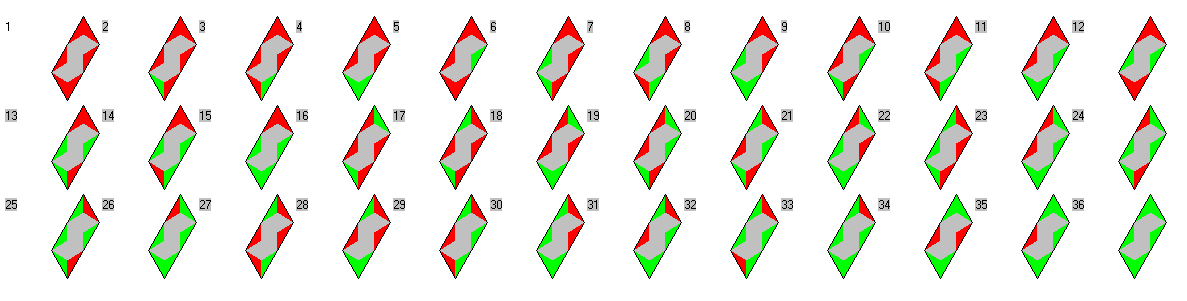With this set you can make parallelograms of size 12x6 and 8x9 with uniformly red colored border.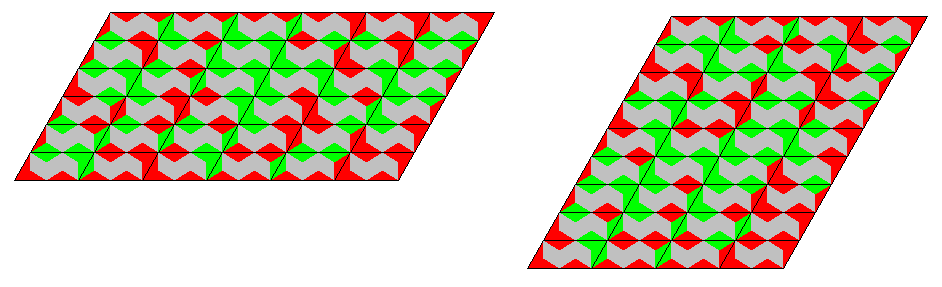The reflected parallelogram can't be constructed because the 9x8 parallelogram has a horizontal side of odd length and for the 6x12 parallelogram there are not enough pieces with red colored short edges. We have 16 pieces with one red colored short edge. But we need 24 edges for the border of the 6x12 parallelogram. If we place some of the 10 pieces with two red colored short edges at the border we need for each two pieces another one to balance the red colored short edges. Therefore we get no more than 6 additional unit edges and 16+6 < 24.

### Two Colors, Two-sided 2x1 Parallelograms

If we are allowed to turn over the pieces we additionally have reflections of the already shown parallelograms. These are parallelograms of size 6x12 and 9x8. Furthermore we can get a parallelogram of size 18x4 and its reflection. A picture of a hexagonal ring is at the beginning of the section.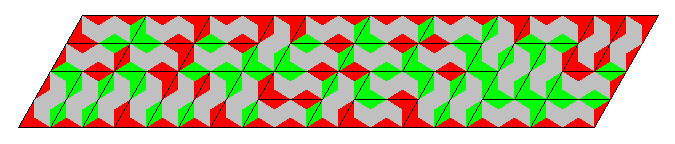### Two Colors, One-sided 2x1 and 1x2 Parallelograms

If it's not allowed to turn over the pieces you can choose the combined set of edge colored 2x1 and 1x2 parallelograms. The picture shows a rhomb of size 12x12, which is constructed with this set. It's the combination of two 6x12 parallelograms. But it isn't possible to use only 2x1 pieces for one parallelogram and 1x2 pieces for the other one.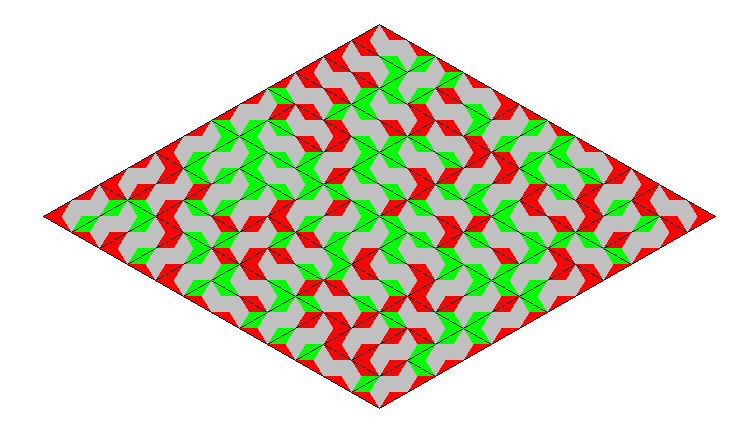The same applies to the three parallelogram of size 6x24, 8x18, 9x16 and their reflections.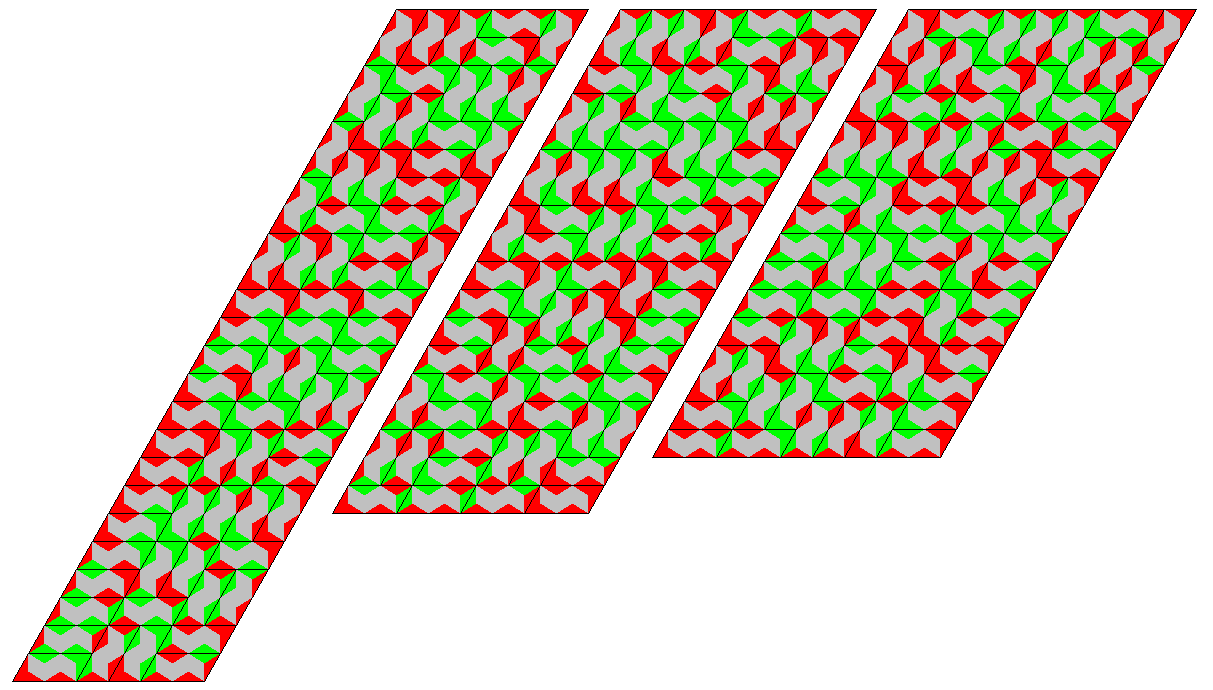For constructions with triangle symmetry I divided the whole figure into three parts. For the left figure the size of the border parallel to the long sides is (12+8)*3=60 unit edges, but the 2-1 and 1-2 pieces can only contribute 30 and 26 unit edges, respectively. Therefore the hole got a green border.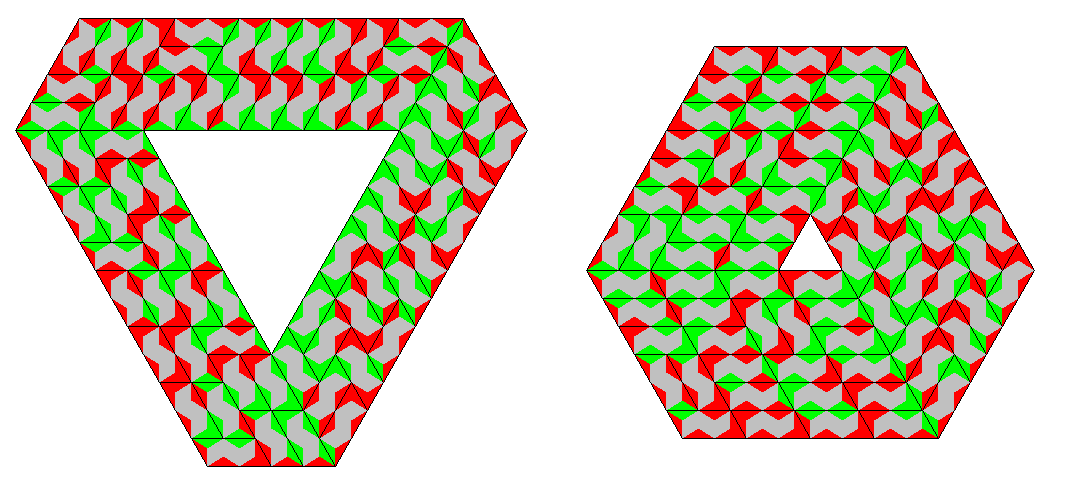Even a figure with hexagonal symmetry can be made.### Three Colors, One-sided 2x1 Parallelograms

There are 378 pieces with an total area of 1512 unit triangles or 756 unit rhombs. Parallelograms of size 54x14,42x18, 18x42 and 14x54 are shown below.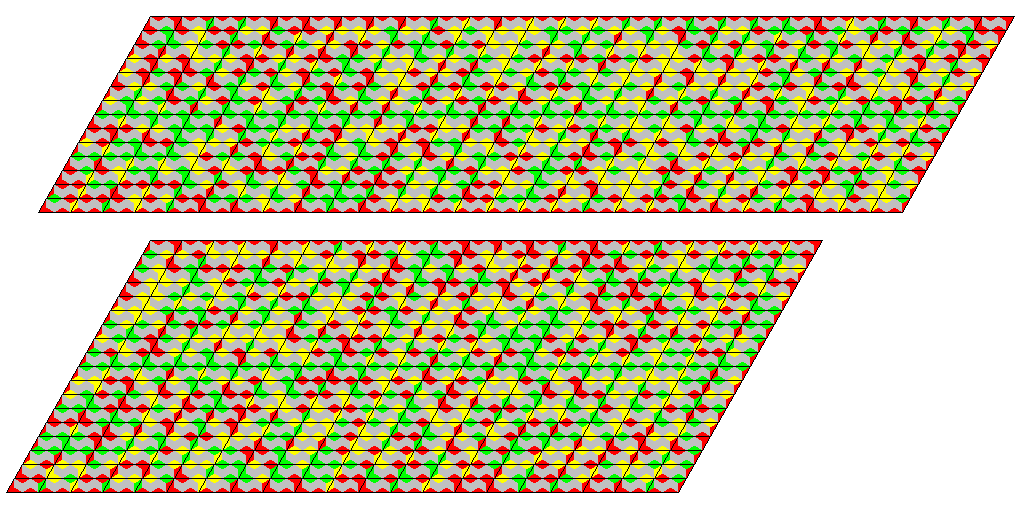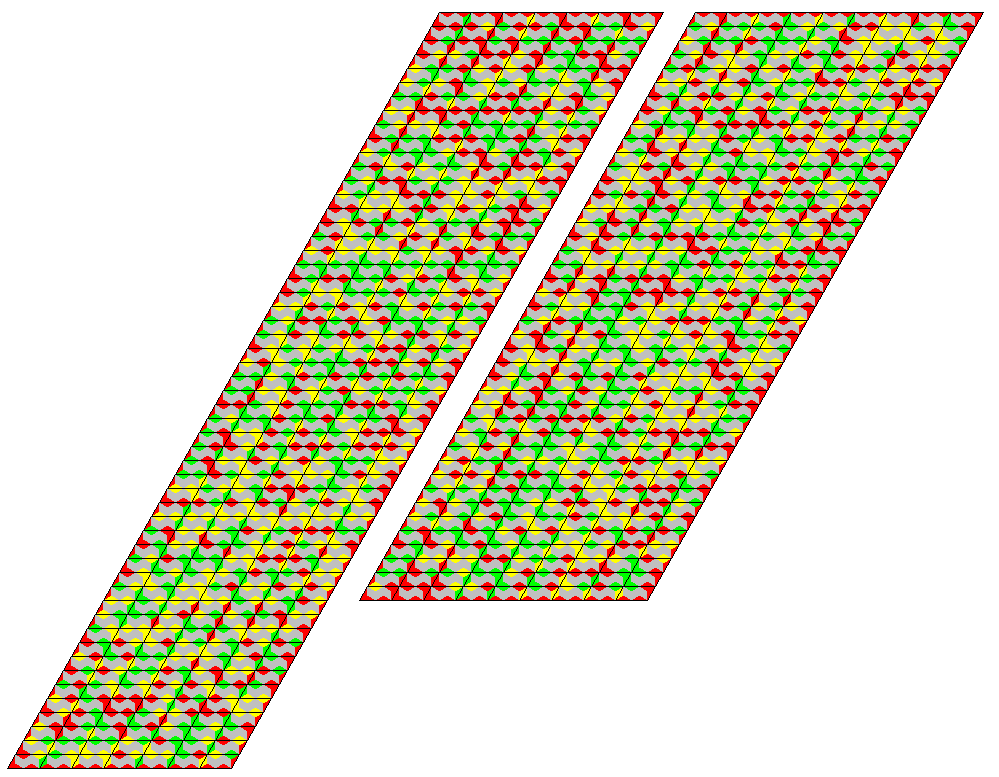Parallelograms of size 36x21, 28x27 and 12x63 are also possible but their reflections have odd horizontal lines, which are not suitable for 2x1 pieces.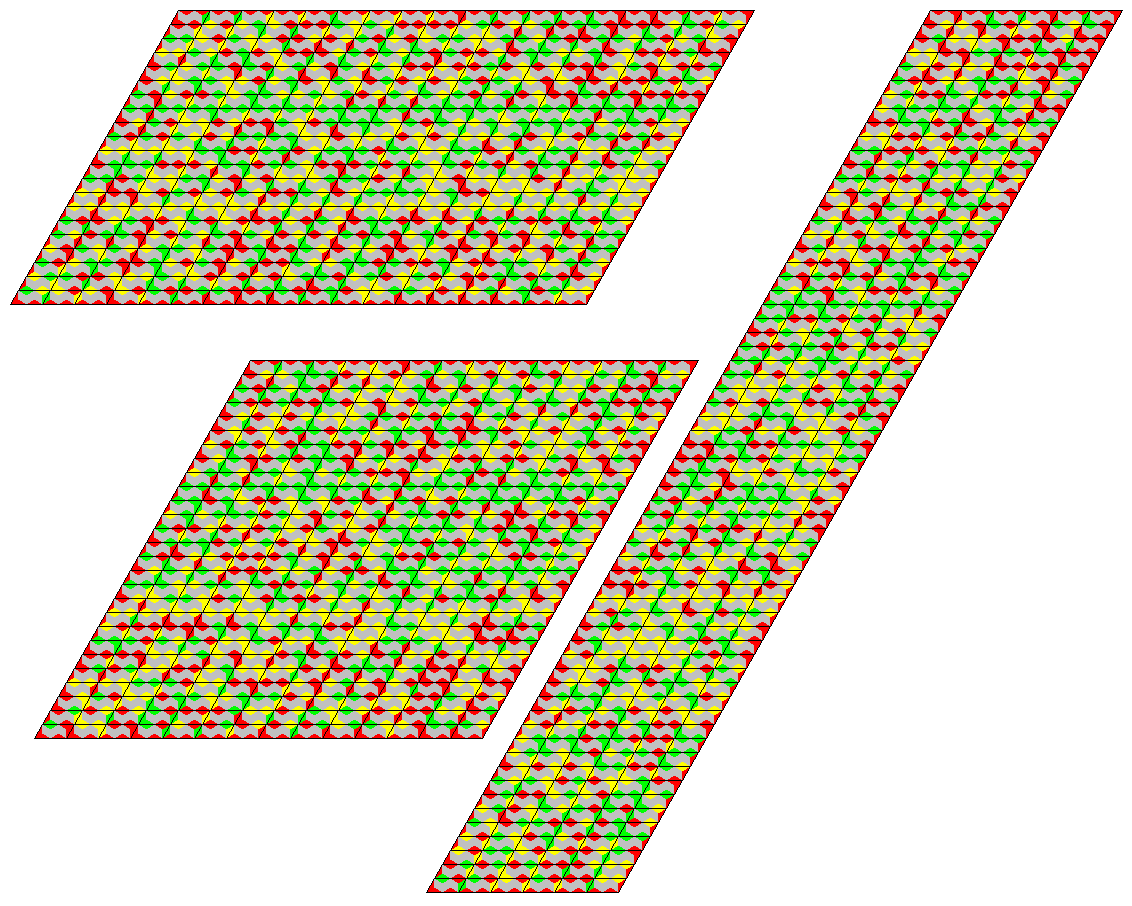The 84x9 parallelogram cannot be constructed. The long sides must be touched by 84 2x1 pieces with red colored long edges, but only 78 pieces are available.
For a hexagon of size16 with a hexagonal hole of size 2 I split the whole figure into 6 parts and preset the colors of the borders. It's important to balance the different color combinations at the borders.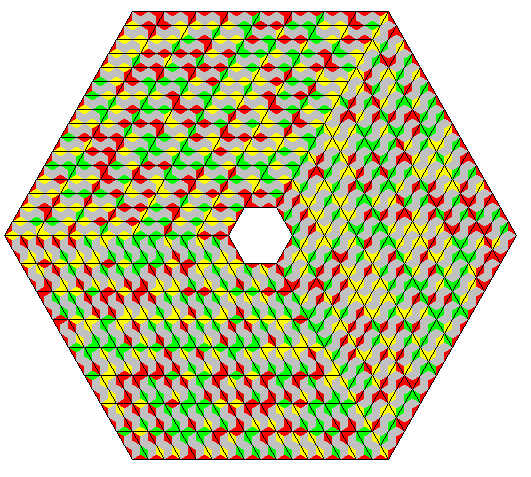3-fold constructions with three different colors at the border are possible, too. If you take a given piece and replace the colors 1,2,3 by 3,1,2 or 2,3,1, you get 126 triples of pieces. If you use only one piece of a triplet for a construction two other constructions are given by a color shift.. This way I made parallelogram of size 42x6, 21x12 and 6x42. Only the items with red borders are shown.### Three Colors, Two-sided 2x1 Parallelograms

With the 2x1 one-sided pieces we can't get the 21x36, 27x28 and 63x12 parallelograms because of the odd horizontal sides. But now we are allowed to turn over the whole constructions of the 36x21, 28x27 and 12x63 parallelograms and have solution for the other ones. To solve the 84x9 parallelogram I split the figure into 28x3 and 28x6 parts, so that one long border is touched by long edges of the pieces and the other one by short edges.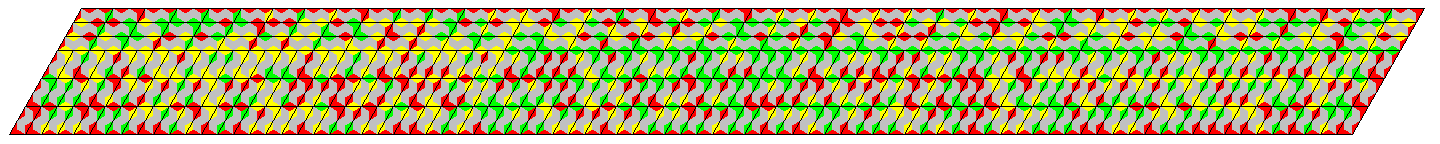### Three Colors, One-sided 2x1 and 1x2 Parallelograms

The combined set of one-sided 2x1 and 1x2 parallelograms has 756 pieces with an area of 1512 unit rhombs. Since the 54x14 and the 14x54 parallelogram can be covered by 2x1 pieces, we can reflect the 14x54 construction and have solutions for the 54x28 and 108x14 parallelograms with the combined set. If we reflect then 54x14 construction we get 14x108 and 28x54 parallelograms.The same applies to the 42x18 and 18x42 parallelograms getting 84x18,18x85,36x42 and 42x36 parallelograms.
For the 24x63 parallelogram this method doesn't work, because the 12x63 parallelogram can only be made by 2x1 pieces but cannot be made by 1x2 pieces. Therefore an alternative partition was used. The following pictures are SVG-graphics to allow for high resolution prints.Instead of searching partitions for the missing parallelograms I decided to look for a hexagonal ring of size 23 with a hole of size 7. The partition was rather difficult because you can't divide the ring into three congruent parts using lines form one outer corner to an inner one. After the inner ring of width 2 was finished I had to preset the border colors to balance the color combinations.Back
Home